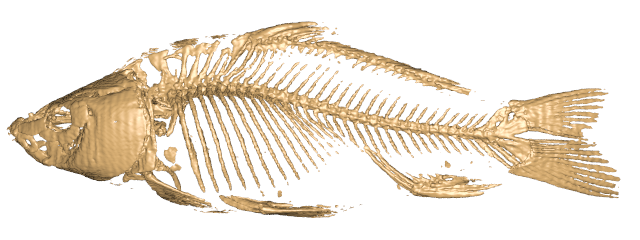# Box Spline Reconstruction On the Face Centered Cubic Lattice

## Minho Kim, Alireza Entezari and Jörg Peters

IEEE Visualization 2008

## (Supplementary page)

How to integrate spline evaluation module into POV-Ray?
- The following process is based on “How to add own internal function” (by Wlodzimierz ABX Skiba). Note that the green parts need to be replaced by the user with proper stuff.
1. Download spline evaluation module (eval_spline_6dir_FCC.cpp) in \$POVRAYSRCPATH\$/source (where fnintern.cpp is located). (\$POVRAYSRCPATH\$/source is the path where the POV-Ray source files are located.)
2. Open the file fnintern.cpp.
3. Add the function declaration at the beginning in the "Global functions" list.
DBL f_eval_spline_6dir_FCC(DBL *ptr, unsigned int fn);
4. Append the function pointer at the end of the array variable POVFPU_TrapTable[]. Note that the position in the array becomes the function id of that function. You can find the index for each function pointers in POVFPU_TrapTable[] at each line. This id will be used later when modifying \$POVRAYSRCPATH\$/include/functions.inc.
{ f_eval_spline_6dir_FCC, 0 + 4 },
5. Increase the value of the variable POVFPU_TrapTableSize by 1.
6. Implement the body of the function DBL f_eval_spline_6dir_FCC(DBL *ptr, unsigned int). Below is an example which loads (only once) the FCC dataset in the global variable g_dataset_fcc. The dataset is assumed to be stored in the absolute path FULL_PATH_WHERE_DATASET_IS_STORED. Various ways of loading dataset is possible.
#include "eval_spline_6dir_FCC.cpp"
TFCC g_dataset_fcc;
DBL f_eval_spline_6dir_FCC(DBL *ptr, unsigned int)
{
double p = {PARAM_X, PARAM_Y, PARAM_Z};
{
{
report_fcc(g_dataset_fcc);
}
else
{
}
}
return eval_spline_6dir_FCC(g_dataset_fcc,p, PARAM(0));
}
7. Now it's time to connect the function id to the actual function name so that the POV-Ray engine can recognize it. Open \$POVRAYSRCPATH\$/include/functions.inc and insert the following where FUNCTION_ID is the function id obtained above.
#declare f_eval_spline_6dir_FCC = function{internal(FUNCTION_ID)};
8. Rebuilt POV-Ray by make install in \$POVRAYSRCPATH\$.
file format (*.fcc)
- Refer to load_fcc() in eval_spline_6dir_FCC.cpp.
 size type description 4byte int version (major) 4byte int version (minor) 4byte int flag (currently used values: FLAG_FCC_PACKED (0x01) and FLAG_FCC_QUASI (0x02)) 8byte double min/max x where the spline is defined 8byte double min/max y where the spline is defined 8byte double min/max z where the spline is defined 12byte double unit length along x,y,z 12byte double inverse of unit length along x,y,z (pre-computed for faster calculation) 16byte int size of the dataset (about half of the values are invalid if FCC_FLAT_PACKED is not set) various double raw dataset
unpacked vs. packed file format

When considering the FCC lattice as a sub-lattice of Cartesian lattice (as integer lattice) such that the sum of coordinates is even, we can store the FCC dataset in a 3D array but ends up wasting about half of the space.

On the other hand, on any all-even coordinate lattice point (2i,2j,2k), which is also an FCC lattice point, we can group four points (2i,2j,2k), (2i,2j+1,2k+1), (2i+1,2j,2k+1) and (2i+1,2j+1,2k) and cover the whole FCC lattice points. In this way we waste only small amount of storage (even no waste in some cases). Therefore, in packed file format, we store the FCC dataset in a 4D array with their indices mapped as follows: (mod and ⌊⋅⌋ are the modulo and floor operators, respectively.)

• if mod((x,y,z),2) == (0,0,0) then (x,y,z) → (⌊x/2⌋,⌊y/2⌋,⌊z/2⌋,0)
• if mod((x,y,z),2) == (0,1,1) then (x,y,z) → (⌊x/2⌋,⌊y/2⌋,⌊z/2⌋,1)
• if mod((x,y,z),2) == (1,0,1) then (x,y,z) → (⌊x/2⌋,⌊y/2⌋,⌊z/2⌋,2)
• if mod((x,y,z),2) == (1,1,0) then (x,y,z) → (⌊x/2⌋,⌊y/2⌋,⌊z/2⌋,3)
file list
• d6_visualize_shifts.m: A MATLAB script that visualizes the shifts (with BB-coefficients) of the 6-direction box spline overlapping two types of polynomial domain.
• eval_spline_6dir_FCC.cpp: C++ source code to evaluate a spline of the 6-direction box spline, i.e., an implementation of Algorithm 2.
Note that no optimization for speed-up is considered in this source code since it is provided as a sample implementation. For practical use, it is recommended to optimize for better evaluation speed.
• dataset.fcc.tgz: Sample FCC dataset (compressed).
• carp.pov: Test POV file. Try the following:
povray +P +W640 +H240 +A carp.pov
• +P: pause after rendering
• +W640: sets the width to 640
• +H240: sets the height to 240
• +A: turns on anti-aliasing (higher quality but slower rendering)
rendered imageReconstruction Gallery